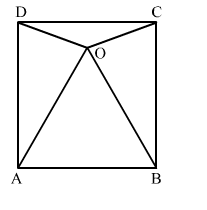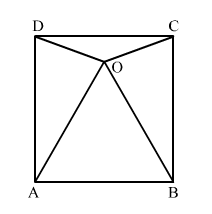# In the given figure, O is a point in the interior of square ABCD such that ΔOAB is an equilateral triangle.

Question:

In the given figure, O is a point in the interior of square ABCD such that ΔOAB is an equilateral triangle. Show that ΔOCD is an isosceles triangle.Solution:Given: In square ABCD, ΔOAB is an equilateral triangle.

To prove: ΔOCD is an isosceles triangle.

Proof:

$\because \angle D A B=\angle C B A=90^{\circ} \quad$ (Angles of square $A B C D$ )

And, $\angle O A B=O B A=60^{\circ}$  (Angles of equilateral $\triangle O A B$ )

$\therefore \angle D A B-\angle O A B=\angle C B A-\angle O B A=90^{\circ}-60^{\circ}$

$\Rightarrow \angle O A D=\angle O B C=30^{\circ} \quad \ldots \ldots$ (i)

Now, in ΔDAO and ΔCBO,

AD = BC                   (Sides of square ABCD)
">DAO = ">CBO      [From (i)]
AO = BO                  (Sides of equilateral ΔOAB)

"> By SAS congruence criteria,
ΔDAO "> ΔCBO

So, OD = OC         (CPCT)
Hence, ΔOCD is an isosceles triangle.